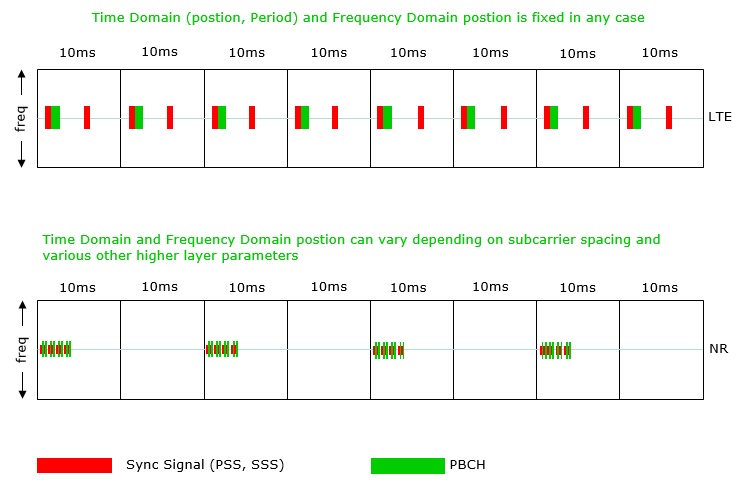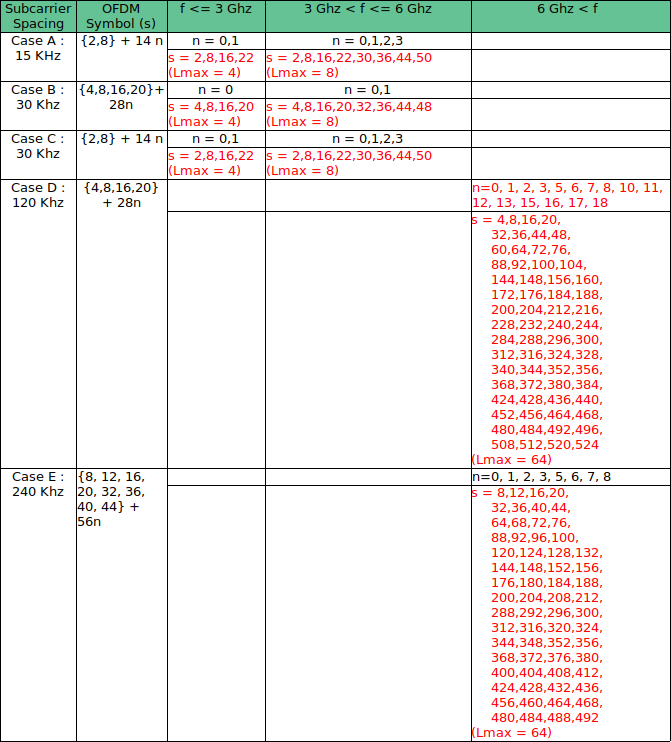### 5G-NR: Synchronisation Signal Block(SSB/SS-Block)

Synchronisation Signal Block(SSB/SS-Block)

3gpp technical specification:

• 38.213
• 38.211
• 38.214
• 38.101
• 38.102
In this blog, we will discuss 5G-NR Synchronization signal block. here we have all the SSB related stubs on one place.

During cell search procedure Synchronization Signal (SS)/ Physical Broadcast Channel (PBCH) Blocks are used. where UE search for the synchronization Signals for getting a cell information to get attach with that cell and access the radio network services.

Before proceeding to decode the system information messages which are transmitted on the PDSCH channel in downlink, UE must decode the PBCH. SS Blocks (SSB) are also used for RSRP, RSRQ and SINR measurements.1- In time domain, it consist of 4 OFDM symbols,  and in Freq domain it consists of 20 RBs. (20x12 = 240 sub carrier) , 1RB = 12 subcarrier

OFDM symbol 0 = PSS (sub carrier  range from 56 to 182)
OFDM symbol 1 = PBCH (sub carrier  range from 0 to 230)
OFDM symbol 2 = SSS (sub carrier  range from 56 to 182)
symbol 2 = PBCH(0 to 47 and 192 to 239)
OFDM symbol 3 = PBCH (sub carrier  range from 0 to 230)

In LTE, the position of Syncronization signal is  straight forward located around DC carrier (Center 6 PRBS/72 subcarriers).

But in 5G, position of Synchronization signals not fixed and can be located any where across the Carrier Bandwidth.In time domain the first symbol Position is determined by combination of Carrier Bandwidth (CBW) + Sub-carrier spacing (SCS).

SSB time domain resourse allocation:

Start symbol of SS block in respect of sub-carrier spacing.In the SA mode, the location of the SSB needs to be obtained through cell search.

SSB Frequency Domain Resources

In NSA mode operation, The location of SSB is determined by information provided by higher layer in RRC Reconfiguration message from eNB to UE, fields are highlighted in below snap.
Under recongigure with sync, below IEs are present.Following information can be extracted from above RRC Message.

• Band = n78 is a TDD band known as TD3500 its frequency range is 3300MHz to 3800 MHz belongs to FR1
• SCS = 30Khz with Carrier Bandwidth= 51 RB’s which is 20 MHz with reference to following figure.FREF = FREF-Offs + ΔFGlobal (NREF – NREF-Offs)                   (i)

• absoluteFrequencyPointA: It represents the common reference point A, this reference point is the 0th RB of 273 RBs, which is the center point of RB#0. From above logs absoluteFrequencyPointA= 642722 (NREF)
• absoluteFrequencyPointA= 3000 MHz + 15* (642722 -600000) KHz= 3,640.83 MHz
• absoluteFrequencySSB: It represent the center frequency of SSB Block. A SSB block is 20 RBs results 20 * 12 =240 Subcarriers and from above logs snippet absoluteFrequencySSB= 643008 (NREF)
• absoluteFrequencySSB= 3000 MHz + 15* (643008 -600000) KHz= 3,645.12 MHz
• Carrier Center Frequency: The total number of RB’s=51 and Resource Block corresponding to center frequency is 51/2=26
• Center Frequency= absoluteFrequencyPointA + 26 RBs * 12 * Subcarrier Spacing
• Center Frequency= 3,640.83 + 26 * 12 *30 KHz = 3,650.19 MHz

Position of SSB from Point A

• offsetToPointA= It defines the frequency offset between point A and the lowest subcarrier of the RB overlapping with SSB. The unit for RB is expressed as 15KHz for FR1 and 60 KHz for FR2
• Kssb= it defines the frequency of RB#0 of SSB and The unit for RB is expressed as 15KHz for FR1 and 60 KHz for FR2

Frequency offset to SSB from Point A = offsetToPointA + Kssb

• Difference between the SSB center frequency (absoluteFrequencySSB) and point A (absoluteFrequencyPointA)
• 3,645.12 3,640.83 = 4290 KHz
• Difference between the Point A and 0th subcarrier RB#0 of SSB
• 4290 – 10 (bottom 10 RB of SSB)* 12 *30 = 690 KHz
• Calculating No. of RBs= 690/180 =3.8
• offsetToPointA = 3
• Kssb = (690 – 3 * 12 *15) / 15 KHz = 10 Subcarriers
• offset to SSB from Point A = offsetToPointA + Kssb = 3 RBs + 10 Subcarrierwhen the network is not using beam forming, it may transmit only one SSB and hence there can only one SSB starting position.